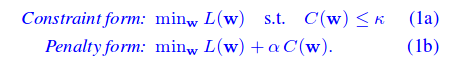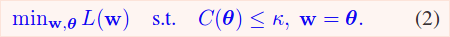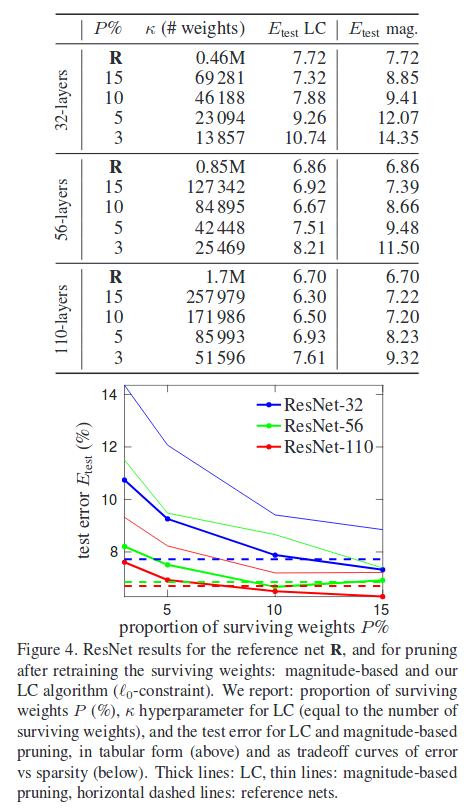The authors propose a method for obtaining resource-efficient neural networks. This method allows to specify a constraint, such as respecting a neuron budget. The method is inspired by the Method of Auxiliary Coordinates (see my other post on that paper).

# Neural network pruning as an optimization problem

The authors study 3 pruning costs: C(w) = ||w||_p where p ∈ [0, 1, 2]. These costs are used with 2 different approaches: the constraint form and the penalty form.## Constraint form

$$w$$ are the trainable weights of the network, and $$\theta$$ is a set of trainable parameters where each parameter is associated with one in $$w$$.

• $$w$$ is trained to lower the cross entropy loss
• $$\theta$$ is optimized to respect the pruning constraint
• $$w, \theta$$ are optimized to be equalAlterning optimization is used. There is a Learning step and a Compression step. In the learning step, we minimize the following:

$L(w) + \frac{\mu}{2} |w - \theta|^2$

We will drive $$\mu \to \infty$$. In the compression step, we minimize

||w - \theta||^2 s.t. C(\theta) < \kappa.

In practice, the Compression step is done algorithmically by keeping the top-$$\kappa$$ weights in $$\theta$$ and zeroing-out the rest (remember that only $$\theta$$ is changed here, not $$w$$). See paper for all the crunchy details, formulas, theorems and proofs.

## Penalty form

The penalty form is very similar and is proved to be equivalent. The Learning step is the same, and the Compression step replaces the constraint with a term to minimize.

## Global vs. Local sparsity

With this method, it is possible to find networks respecting a budget with different compression ratios for each layer, and so naturally.

# Results

Results are pretty goodGood luck.